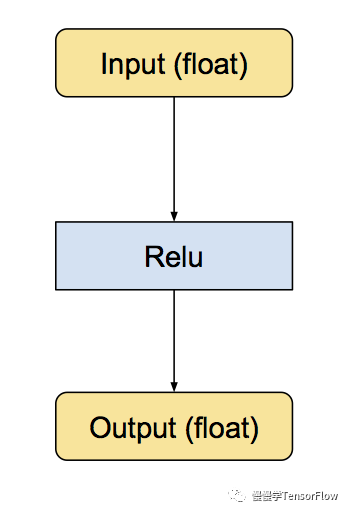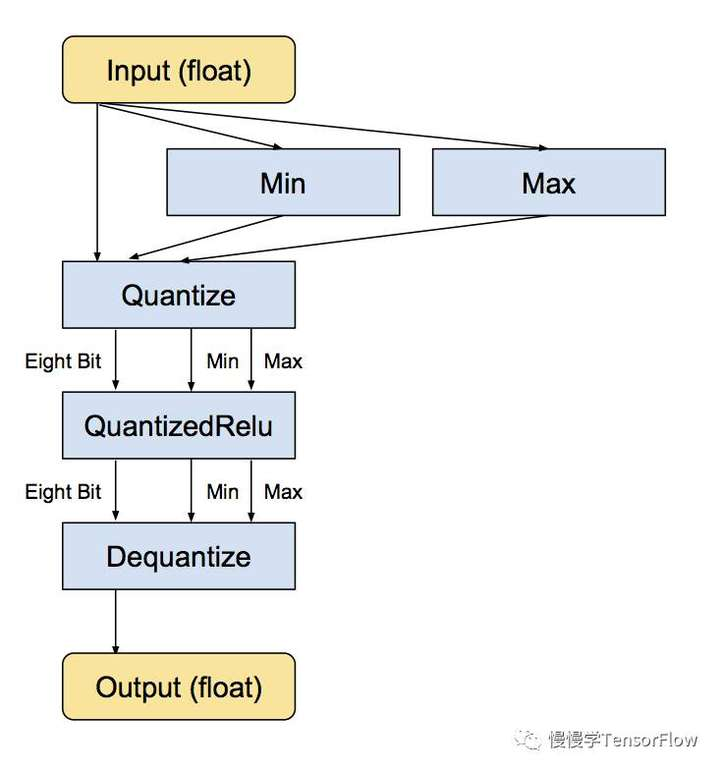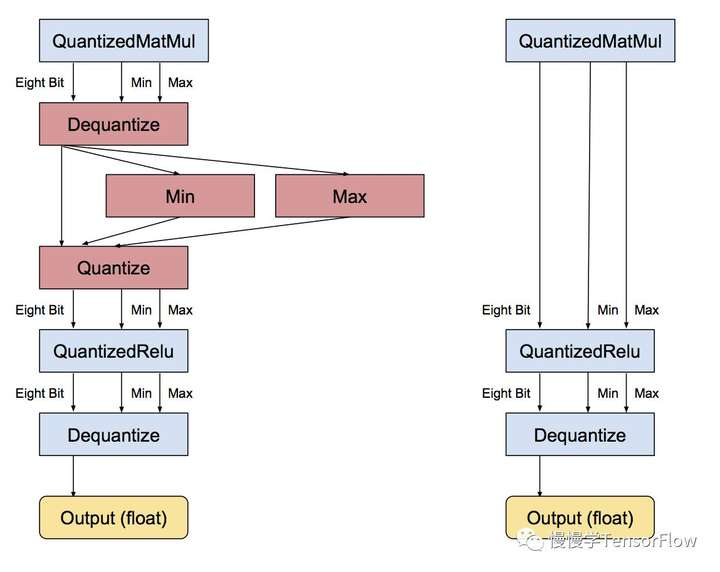# 量化神经网络

TensorFlow 在产品级别内在支持 8-bit 计算。它也有一个将用浮点训练好的模型转换为等效的利用量化算法进行预测的图。

$curl download.tensorflow.org -o /tmp/inceptionv3.tgz$ tar xzf /tmp/inceptionv3.tgz -C /tmp/

$cd ~/tensorflow-1.0.0-rc1/$ bazel build tensorflow/tools/quantization:quantize_graph

$bazel-bin/tensorflow/tools/quantization/quantize_graph \ --input=/tmp/classify_image_graph_def.pb \ --output_node_names="softmax" \ --output=/tmp/quantized_graph.pb \ --mode=eightbit 运行截图见下图所示：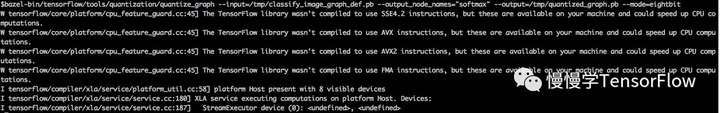这样将会产生一个新模型 quantized_graph.pb，与原始模型运行相同计算，但内部使用 8-bit 计算，所有权值也做 8-bit 量化。 如果你注意文件尺寸，会发现它只有原先模型的 1/4 （ 23MB vs 91 MB）。 你仍然可以运行这个模型使用几乎相同输入和输出，并获得等价的结果。下面是例子：$ bazel build tensorflow/examples/label_image:label_image

\$ bazel-bin/tensorflow/examples/label_image/label_image \

--image=<input-image> \

--graph=/tmp/quantized_graph.pb \

--labels=/tmp/imagenet_synset_to_human_label_map.txt \

--input_width=299 \

--input_height=299 \

--input_mean=128 \

--input_std=128 \

--input_layer="Mul:0" \

--output_layer="softmax:0"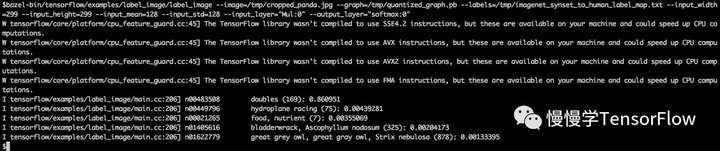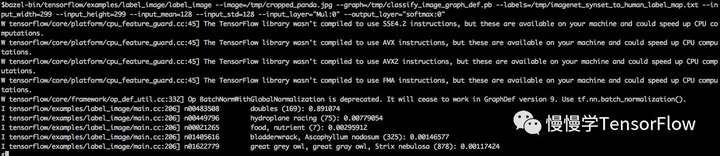TF 已经通过编写等价的预测时常用的 8-bit 版本运算符实现了量化计算，这些运算符包括卷积、矩阵乘、激活函数、下采样和拼接。转换脚本首先替换所有已知的运算符为等价的量化运算符。